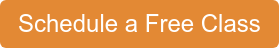If you were to ask your 5th grader "What's scarier: the bogeyman or fractions?", chances are, they would choose the latter. Fractions are scary: they are an entirely new concept and they can be very difficult to understand, initially.

Fractions are like the troll in front of the bridge; the dragon guarding the castle. They are a difficult ordeal -- but they must be overcome. And truth be told, fractions are quite easy to overcome... we just need to start at the beginning!

## What Are Fractions?

Fractions are just breaking something whole into smaller, equal parts. From a quarter tank of gas to half a pie of pizza, there are real world examples all around you. So try and start by bringing this 'real-world' awareness to your child, and then build from there.

## The Parts of a Fraction

The bottom number of a fraction is called the denominator. The denominator tells us how many parts the whole is divided into! (For example, if we have a pizza-pie with 8 slices, the denominator of our fraction would be 8)

The top part of the fraction is is called the numerator. The numerator tells us how many parts of the whole we have! (For example, if I am really hungry and take 5 slices from the pizza pie, the numerator would be 5).See? Easy as pie :)

The first and most important step is having a common denominator.
"BUT WHY DO WE HAVE TO HAVE A COMMON DENOMINATOR! WHY CAN'T I JUST ADD 3/4 + 2/3??"

Don't let this question frustrate you -- it's completely valid! Why do we need a common denominator? When we add 'normal' numbers we don't have to worry about a common denominator...

Well think of it this way: a different denominator means a completely different type of a fraction:

'one-fourth' is different from 'one-third' in the same way 1 apple is different from 1 orange!

So in the same way, you can't add 2 oranges to 3 apples and say you have 5 orapples, you just can't add 3/4 to 2/3 -- they're two completely different things!

Remind your kid, just like you make sure you're only counting apples when asked to add apples, make sure you have a common denominator. (Yes, they'll complain -- but maybe one day they'll stop... probably when they're teaching their own kids!)

1. Make sure the bottom numbers (the denominators) are the same.
2. Add or subtract the top numbers (the numerators), put that answer over the denominator.
3. Simplify the fraction (if possible)

## Multiplying Fractions

When compared to adding or subtracting fractions, multiplying fractions really is easy as pie!## Dividing Fractions

Dividing fractions can seem daunting, but it's quite easy to do, if you remember the trick: Keep, Change, Flip!

1. Keep the first fraction the same
2. Change the division sign to a multiplication sign
3. Flip the last fraction
4. Multiply, and you're done!Overwhelmed? Don't worry, fractions are a scary monster for any kid (or anyone who has to teach them fractions, for that matter). Here at Math Genie, we've made it our job to break down such complex problems into simple concepts. We want your child to understand not only how to do something, but also why they are even doing it. Why? Well, it's scientifically proven! When we understand why, we remember it better!

Come try a free class and see if we can help your child slay the fractions monster by understanding all the tricks and spells they have in that wonderful little brain of theirs!### GET A FREE CLASS